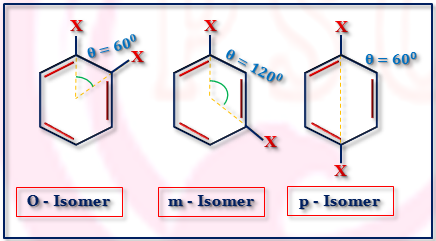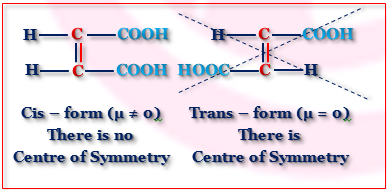# Application of Dipole Moment for Molecules

### Dipole moment of benzene and its derivatives

Zero dipole moment of the benzene suggested that it has regular hexagonal planer structure and it confirms Kekule’s form.Regular Planer Hexagonal Structure of Benzene having Centre of Symmetry
However, if hydrogen atom is substituted by another atom or group, it acquires polar character.
Examples of such derivative of benzene are C₆H₅Cl, C₆H₅NO₂, C₆H₅OH, etc.
When di-substituted benzene are considered it can be shown that o-isomer will have the highest value of dipole moment then other two isomers. p-derivative has the lowest value while m-derivative has the value between the two.
The values can be calculated using the vector addition principle
μ = m12 + m22 + 2 m1m2 cosθ
provided the group must also lie on the same plane of the benzene ring.

### Di-substituted derivative of benzene:Di-substituted derivative of benzene
 μ² = m12 + m22 + 2 m1m2 cosθHere, m1 = m2 = m and θ = 60°Thus, μ² = 2 m²(1 + cos60°)  = 2 m² (1 + 1/2) ∴ μ= √3 m
 μ² = m12 + m22 + 2 m1m2 cosθHere, m1 = m₂ = m and θ = 120°Thus, μ² = 2 m²(1 + cos120°)  = 2 m² (1 - 1/2)∴ μ = m
 μ² = m12 + m22 + 2 m1m₂ cosθHere, m1 = m2= m and θ = 180°Thus, μ² = 2 m²(1 + cos180°)  = 2 m² (1 - 1)∴ μ = 0
Thus μ of o-isomer〉
μ of m-isomer〉μ of p-isomer
(this has been confirmed for C₆H₄Cl₂ and C₆H₄(NO₂)₂.
This determination of dipole moment helps to determine the orientation of the groups in the benzene ring.
• Problem 1:
p - dinitro benzene has μ = 0 but p - dihydroxy benzene μ ≠ 0. Explain.
Through the p-dinitro benzene has μ = 0. p-dihydroxy benzene has dipole moment(μ ≠ 0). This is due to the fact that the two substituted hydroxy group are not on the same plane of the benzene ring but are inclined to the ring.

### (v) Cis and Trans forms of Geometrical Isomerism:

Presence of double bond in C - C link restricts the free rotation and geometrical isomerism develops.Dipole Moment of Cis and Trans Isomers
The Measurement of dipole moment helps to distinguish the two forms of geometrical isomers.

### (vi) Change of Dipole Moment with Temperature:

For molecule CH₂Cl - CH₂Cl, several confirmations are also changed and so dipole moment (μ) of the molecule is also changed.
• Problem 2:
The dipole moment of o-xylene = 0.693 D. Finds the dipole moment of toluene.
For o - xylene the θ = 60° and μ = √3 m. Thus, m = 0.693/√3 = 0.4 D
Again θ = 120° for toluene and dipole moment(μ) of toluene = m.
Thus the dipole moment of toluene is 0.4 D.

How to Calculate Dipole Moment of a Compound ? (i) For Penta-atomic Molecules(AX₄), (ii) Hepta-Atomic Molecules( AX₅), (iii) Benzene and its Derivatives.

Name

Email *

Message *